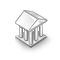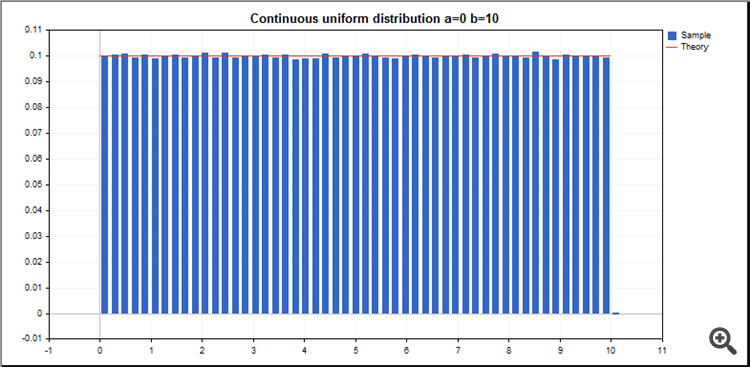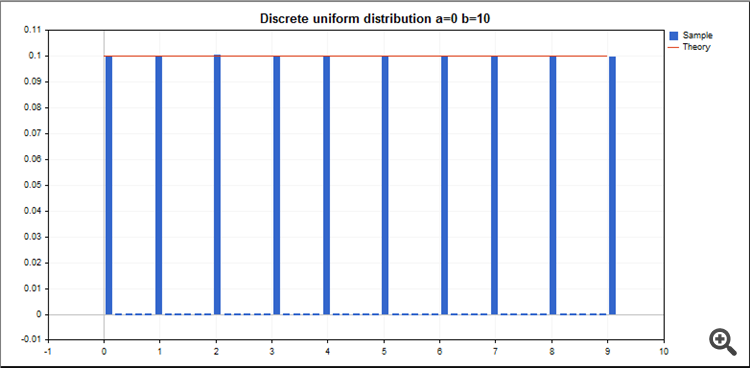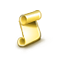Interesting script?
So post a link to it -
let others appraise it

You liked the script? Try it in the MetaTrader 5 terminal# Class CRandom - library for MetaTrader 5

Views:
1210
Rating:
Published:
2019.06.16 06:20
Updated:
2019.06.26 08:03

### MathRand() in MQL

The standard random number generator of mql has a fairly limited number of possible values from 0 to 32767 (15-bits usable, 2^15 = 32,768 values).

PCG is a family of random number generators, which are fast, statistically excellent, and have small code size.

### 32-bits PCG Generator

This class is a wrapper class around the high-quality 32-bits PCG generator. This RNG has an output range of 2^32 which means it can generate 4,294,967,296 possible values.

A permuted congruential generator (PCG) is a pseudorandom number generation algorithm developed in 2014 which applies an output transformation (random shift or rotation) to eliminate the short period problem in the low-order bits that other LCG generators suffer from. It achieves excellent statistical performance with small and fast code, and small state size.

The class provides an easy interface for random number generation.

```//+------------------------------------------------------------------+
//| Class CRandom                                                    |
//| Usage: Random number generation using the 32-bit PCG generator.  |
//+------------------------------------------------------------------+
class CRandom
{
public:
int          RandomInteger(const int max = INT_MAX);              // Random integer [0,max) - default [0,2147483647)
int          RandomInteger(const int min,const int max);          // Random integer [min,max)

double       RandomDouble(void);                                  // Random double  [0,1)
double       RandomDouble(const double min,const double max);     // Random double  [min,max)

bool         RandomBoolean(void);                                 // Random true/false (equal probability)
bool         RandomBoolean(const double prob_true);               // Random true/false (with prob_true)

double       RandomNormal(void);                                  // Random double (normal distribution)
double       RandomNormal(const double mu,const double sigma);    // Random double (normal distribution)
};
```

This GRAPH was obtained for random double numbers from 0 to 10And, that one for random integer numbers from 0 to 10The BITMAP of the low-order bits shows also a random patternGbpChfArrowDrawingIndiEuSmoothed CCIRSI candles - lite ressource